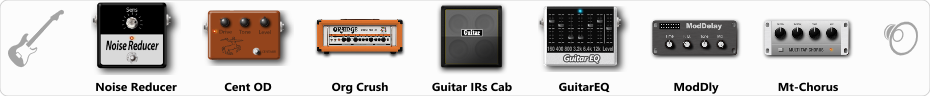# Hard Rock FX

Discussion in 'ToneLib-GFX presets' started by Savandil, Aug 18, 2019.

1. Hard Rock FX

Preset name: Hard Rock Dly&Chr

Effects chain:Effect: "Noise Reducer" (Dynamics / Filter), active - "yes"
"Sens" = 60
"Mode" = Soft

Effect: "Cent OD" (Overdrive / Distortion), active - "yes"
"Drive" = 38
"Tone" = 76
"Level" = 70

Effect: "Org Crush" (Amp simulators), active - "yes"
"Gain" = 35
"Bass" = 70
"Middle" = 65
"Treble" = 40
"Presence" = 60
"Master" = 60
"Output" = 50
"Level (dB)" = 0

Effect: "Guitar IRs Cab" (Cabinets), active - "yes"
"Model" = Orange PPC (4x12")
"Mic Position" = Center
"Mic Distance" = Near
"Low Cut (Hz)" = 120
"Hi Cut (kHz)" = 12.5
"Mix" = 100
"Level (dB)" = 0

Effect: "GuitarEQ" (Dynamics / Filter), active - "yes"
"160 Hz" = -2
"400 Hz" = 0
"800 Hz" = -2
"1.6 kHz" = 0
"3.2 kHz" = -2
"6.4 kHz" = -3
"12 kHz" = 3
"Level (dB)" = 0

Effect: "ModDly" (Delay), active - "yes"
"Time" = 457
"Feedback" = 30
"Tone" = 70
"Speed" = 1.6
"Mix" = 50

Effect: "Mt-Chorus" (Modulation / Sfx), active - "yes"
"Speed" = 5.0
"Depth" = 80
"Time" = 1.5
"Mix" = 45

Note: You will need to download and install the ToneLib-GFX software to use the preset.

File size:
617 bytes
Views:
3,211
File size:
617 bytes
Views:
44
File size:
617 bytes
Views:
48
File size:
617 bytes
Views:
39